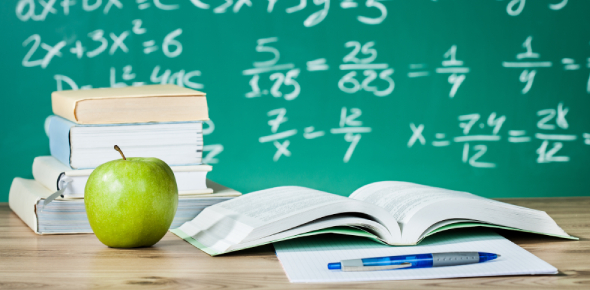# Take The 9th Grade Math Practice Questions!

14 Questions | Total Attempts: 3171Settings.

• 1.
As a decimal 4.5% equals :
• A.

45.0

• B.

4.5

• C.

0.45

• D.

.045

• 2.
0.3% equals
• A.

1/3

• B.

3/1000

• C.

3/100

• D.

3/10

• 3.
A test score of 16 marks out of 64 is what as a percentage?
• A.

20%

• B.

24%

• C.

25%

• D.

30%

• 4.
Of the 510 students at LSC, 90% can swim. How many students can swim?
• A.

500

• B.

459

• C.

420

• D.

51

• 5.
If \$250 is increased by 8%, then the new price is :
• A.

\$230

• B.

\$258

• C.

\$270

• D.

\$242

• 6.
A t-shirt costs \$20. It is on sale for 15% off. How much would you save if you bought it?
• A.

\$15

• B.

\$3

• C.

\$17

• D.

\$5

• 7.
A real estate agent sells a house for \$420,000. If they earn 2.5% commission for every dollar on the sale, how much commission does the agent earn?
• A.

\$15,500

• B.

\$5,000

• C.

\$10,500

• D.

\$5,500

• 8.
A real estate agent sells a house for \$420,000. If they earn 2.5% commission for every dollar OVER \$200,000 on the sale, how much commission does the agent earn?
• A.

\$15,500

• B.

\$5,000

• C.

\$10,500

• D.

\$5,500

• 9.
A PS3 console is advertised at an 8.5% discount. if the price was \$400, what is the discounted price?
• A.

\$366

• B.

\$434

• C.

\$315

• D.

\$360

• 10.
An item is bought for \$80 and sold for \$120. Find the percentage profit on the cost price.
• A.

50%

• B.

30%

• C.

60%

• D.

40%

• 11.
Ted pays \$60 after receiving a discount of 20%. the original price was :
• A.

\$63

• B.

\$75

• C.

\$72

• D.

\$80

• 12.
12.5% of 160 is closest to :
• A.

18

• B.

20

• C.

2.5

• D.

250

• 13.
The Simple Interest earned on \$2000 invested at a rate of 5.5% p.a. for 2 years is :
• A.

55

• B.

110

• C.

220

• D.

200

• 14.
12.5% of the students scheduled for a class turned up - only 20 students arrived!!. How many students would have attended if 100% of the class had managed to get there?
• A.

160

• B.

200

• C.

2.5

• D.

250

Related TopicsBack to top